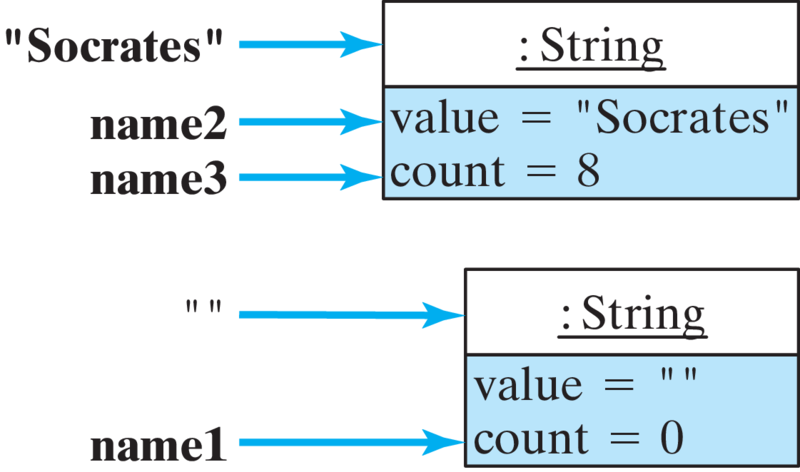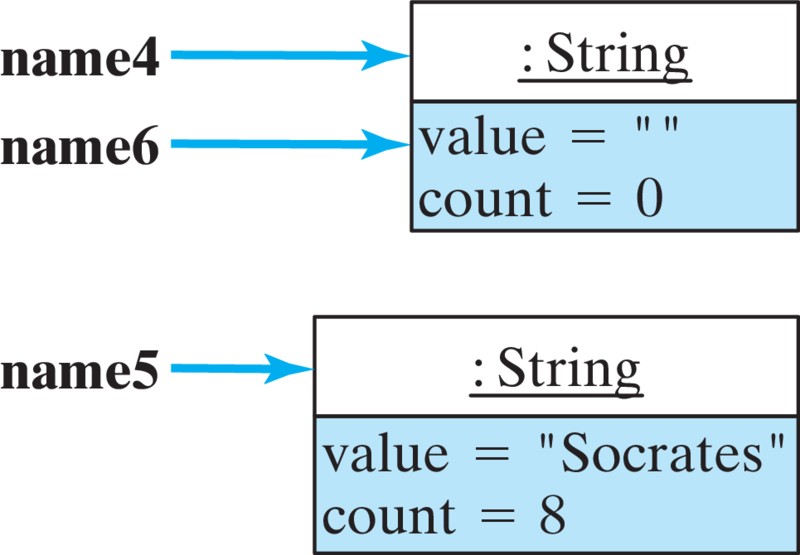# Java, Java, Java: Object-Oriented Problem Solving, 2022E

## Section7.2String Basics

To review, in Java, strings are considered full-fledged objects. A String is a sequence of the characters (data) plus the actions (methods) that are used to manipulate the string. The java.lang.String class (Figure 7.2.1) is a direct subclass of Object, and it contains many public methods that can be used to perform useful operations on strings (such as concatenation).
We will discuss a selection of the more commonly used methods, but for a full description of the String class see the official Java documentation.
Like other object variables, String variables serve as references to their respective objects. However, unlike other Java objects, the String type has certain characteristics in common with the primitive data types.
For example, as we have already seen, Java allows for literal strings. A string literal is a sequence of zero or more characters contained in double quotes, such as “Socrates” and “” (the empty string). Java allows us to perform operations on literal strings, such as concatenation. As we have already seen, the expression "Hello" + "world" results in the string "Helloworld". Java also allows us to use string literals to initialize String variables with an assignment statement.
Given how much we use String objects, these exceptional features represent a good design decision for Java.

### Subsection7.2.1Constructing Strings

To create String objects, the String class provides many constructors, including the following:
public String();        // Creates an empty string
// Copy constructor: Creates a copy of a string
public String(String initial_value);

When we create an object using the default constructor, as in
String name = new String();

Java will create a String object and make name the reference to it (e.g., Figure 7.2.2). In addition to storing the sequence of characters that make up the string, Java also stores an integer value representing the number of characters in the string. For the default constructor, the object’s value is the empty string and its count is 0.
Although we have chosen to represent these two elements as the instance variables, value and count, we don’t know exactly how Java stores the sequence of characters. That information is hidden.
The second constructor in Figure 7.2.1 is the copy constructor, used to clone or duplicate an object. Many Java classes have copy constructors. Consider the following statements:
String s1 = new String("Hello");
String s2 = new String(s1);

These two statements would result in two distinct String objects, both storing the word “Hello”.
Note that in the first of the preceding statements, we used the literal string “Hello” in the constructor.
When Java encounters a new literal string in a program, it constructs an object for it. For example, if your program contained the literal “Socrates,” Java would create an object for it and treat the literal itself as a reference to the object (Figure 7.2.3).
We often use a string literal to assign a value to a String variable:
String s;      // The value of s is initially null
s = "Socrates";// s now refers to "Socrates" object

In this case, the reference variable s is initially null — that is, it has no referent, no object, to refer to. However, after the assignment statement, s would refer to the literal object “Socrates,” which is depicted in Figure 7.2.3. Given these two statements, we still have only one object, the String object containing the word “Socrates”. But now we have two references to it: the literal string “Socrates,” and the reference variable s.
Assignment statements can also be used as initializers when declaring a String variable:
String name1 = "";         // Reference to the empty string
String name2 = "Socrates"; // References to "Socrates"
String name3 = "Socrates";

In this example, Java does not construct new String objects. Instead, as Figure 7.2.4 shows, it simply makes the variables name1, name2, and name3 serve as references to the same objects that are referred to by the literal strings “” and “Socrates.”
This is a direct consequence of Java’s policy of creating only one object to serve as the referent of a literal string, no matter how many occurrences there are of that literal in the program. Thus, these declarations result in no new objects, just new references to existing objects. By npt duplicating lierals, this policy saves lots of memory in our programs.Figure 7.2.4. The variables name1, name2, and name3 serve as references to the literals “Socrates” and ’’.
Finally, consider the following declarations, which do invoke the String constructors:
String name4 = new String();    // Creates an object
String name5 = new String("Socrates");
String name6 = name4;

In this case, as shown in Figure 7.2.5, Java creates two new objects and sets name4 to refer to the first and name5 to refer to the second. It gives name4 the empty string as its value, and it gives name5 “Socrates” as its value. But these two objects must be distinguished from the objects corresponding to the literals “” and “Socrates” themselves. The declaration of name6 just creates a second reference to the object referred to by name4.Figure 7.2.5. Together with the objects in Figure 7.2.4, there are now four different String objects with eight different references to them, including the literals “Socrates” and “”.

#### ExercisesSelf-Study Exercises

##### 1.What is printed?
The following code segment will print:
String s1 = "silly";
System.out.println(s1);

##### 2.What is printed?
The following code segment will print:
String s1 = "silly";
String s2 = s1;
System.out.println(s2);

##### 3.Creating an empty string.
Which the following declarations would create an empty string?
• String s = "";
• That’s one way to create an empty string.
• String s = new String();
• That’s one way to create an empty string.
• String s = " ";
• There’s a space between the quotes.
• String s = new String("");
• That’s one way to create an empty string.
##### 4.String objects.
Given the declarations here:
String s1;
String s2 = "Hello";
String s3 = s2;

1. How many distinct String objects are created?
2. How many null variables are declared?
3. How many references are there to the "Hello" litreral?
Hint.
Review the discussion around Figure 7.2.5.

### Subsection7.2.2Concatenating Strings

Another way to build a String object is to concatenate two other strings. Recall from Chapter 2 that there are two ways to perform string concatenation in Java: We can use the concat() method or the concatenation operator, $$+\text{.}$$
String lastName = "Onassis";
String jackie =
new String("Jacqueline " + "Kennedy " + lastName);
System.out.println("Jacqueline".concat(lastName));

The second of these statements uses the concatenation operator, $$+\text{,}$$ to create the String “Jacqueline Kennedy Onassis.” The third statement uses the String method, concat(), to print “JacquelineOnassis.”
Using the + symbol as the string concatenation operator is another example of operator overloading—using the same operator for two or more different operations—which we encountered in Chapter 5.
Note that primitive types are automatically promoted to Strings when they are mixed with concatenation operators. Thus, the statement
System.out.println("The sum of 5 and 5 = "+ (5 + 5));

will print the string “The sum of 5 and 5 = 10.” Note that the integer addition—(5 + 5)—is performed first, before the integer result is converted into a String. If we had left off the parentheses around the addition operation, the second plus sign would also be interpreted as a concatenation operator. Thus,
System.out.println("The concatenation of 5 and 5 = " + 5 + 5);

would print “The concatenation of 5 and 5 = 55.”

#### ExercisesSelf-Study Exercises

##### 1.What is printed?
The following code segment will print:
String s1 = "silly";
String s2 = new String (s1 + " stuff");
System.out.println(s2);

##### 2.Evaluate expressions.
Evaluate the following expressions given the declarations here. Make sure you use quote marks to distinguish strings ("100") from numbers (100).
int M = 5, N = 10;
String s1 = "51", s2 = "75";

1. M + N
2. M + s1
3. s1 + s2

### Subsection7.2.3Indexing Strings

Programmers often need to take strings apart or put them together or rearrange them. Just think of the many word-processing tasks, such as cut and paste, that involve such operations. To help simplify such operations, it is useful to know how many characters a string contains and to number, or index, the characters that make up the string.
The number of characters in a string is called its length. The String instance method, length(), returns an integer that gives the String’s length. For example, consider the following String declarations and the corresponding values of the length() method for each case:
String string1 = "";           string1.length()  ==> 0
String string2 = "Hello";      string2.length()  ==> 5
String string3 = "World";      string3.length()  ==> 5
String string4 = string2 + " "
+ string3;                 string4.length()  ==> 11

The position of a particular character in a string is called its string index.
All Strings in Java are zero indexed — that is, the index of the first character is zero. (Remember, zero indexing is contrasted with unit indexing, in which we start counting at 1.) For example, in “Socrates,” the letter S occurs at index 0, the letter o occurs at index 1, r occurs at index 3, and so on. Thus, the String “Socrates” contains eight characters indexed from 0 to 7 (Figure 7.2.9). Zero indexing is customary in programming languages. We will see other examples of this when we talk about arrays and vectors.

### Subsection7.2.4Converting Data to Strings

The String.valueOf() method is a class method that is used to convert a value of some primitive type into a String object. For example, the expression, String.valueOf(128) converts its int argument to the String “128.”
There are different versions of valueOf(), each of which has the following type of signature:
static public String valueOf(Type);

where Type stands for any primitive data type, including boolean, char, int, double, and so on.
The valueOf() method is most useful for initializing Strings. Because valueOf() is a class method, it can be used as follows to instantiate new String objects:
String number = String.valueOf(128); // Creates "128"
String truth = String.valueOf(true); // Creates "true"
String bee = String.valueOf('B');    // Creates "B"
String pi = String.valueOf(Math.PI);  // Creates "3.14159"

We have already seen that Java automatically promotes primitive type values to String where necessary, so why do we need the valueOf() methods? For example, we can initialize a String to “3.14159” as follows:
String pi = new String(""+Math.PI);// Creates "3.14"

In this case, because it is part of a concatenation expression, the value of Math.PI will automatically be promoted to a String value.
The point of the valueOf() method is twofold. First, it may be the method that the Java compiler relies on to perform string promotions such as this one. Second, using it in a program—even when it is not completely necessary—makes the promotion operation explicit rather than leaving it implicit. This helps to make the code more readable. (Also, see Exercise 7.9.)

#### ExercisesSelf-Study Exercises

##### 1.String.valueOf().
Evaluate each of the following expressions. Use quote marks to distinguish strings ("100") from numbers (100).
1. String.valueOf(45)
2. String.valueOf (128-7)
3. String.valueOf('X')
Hint.
The String.valueOf() method converts its argument into a string.